# Problem 50631. Cosine effect in solar power towers

In Solar thermal power plants with central receiver (https://en.wikipedia.org/wiki/Solar_power_tower) the mirrors (called heliostats) do not aim at the sun (vector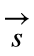) or the tower (vector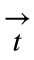), but the bisector of these two directions (vector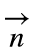):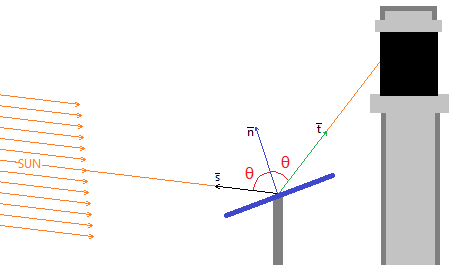The solar irradiance that bounces off the mirror is reduced by an amount proportional to reflectivity (assume 100% for this problem) and to the cosine of the angle between the normal vector to the heliostat and the other vector (sun or tower).
The aim of this problem is to calculate the value of the cosine of the angle (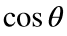) given the normalized vectorsandthat are known for every heliostat at every moment.
Hints:
• Vectors are 3×1, with norm = 1.
• Make use of scalar product to calculate cosines, taking advantage of the normalized vectors.

### Solution Stats

52.94% Correct | 47.06% Incorrect
Last Solution submitted on May 15, 2023

### Community Treasure Hunt

Find the treasures in MATLAB Central and discover how the community can help you!

Start Hunting!